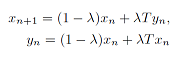##### Exact Explicit Solutions of a Nonlinear Evolution Equation

Zhengyong Ouyang, Shan Zheng

Journal of Advances in Mathematics and Computer Science, Page 308-318
DOI: 10.9734/BJMCS/2015/15751

We employ bifurcation method of dynamical systems to investigate exact traveling wave solutions of a nonlinear evolution equation. We obtain some exact explicit expressions of solitary wave solutions and some new exact periodic wave solutions in parameter forms of Jacobian elliptic function. We point out that the solitary waves are limits of the periodic waves in some sense, the results infer that the periodic waves degenerate solitary waves in some conditions.

##### Resultant-Based Method for Overdetermined Strata for Degree 4 hyperbolic Polynomials

Journal of Advances in Mathematics and Computer Science, Page 319-327
DOI: 10.9734/BJMCS/2015/15865

We present a resultant-based method to calculate the overdetermined strata for degree 4 hyperbolic polynomials in one variable. It is a new method to calculate overdetermined strata. We present also the complete study of the overdetermined strata for degree 4 hyperbolic polynomials by the geometric method.

##### On Existence of Solution for Higher-order Fractional Differential Inclusions with Anti-periodic Type Boundary Conditions

Mohammed M. Matar, Fayez A. El-Bohisie

Journal of Advances in Mathematics and Computer Science, Page 328-340
DOI: 10.9734/BJMCS/2015/15367

This paper investigates the existence of solutions for fractional differential inclusions of order q ∈ (4, 5) with anti-periodic type boundary conditions by means of some standard fixed point theorems for inclusions. The existence results are established for convex as well as the nonconvex multivalued maps. Some illustrative examples are introduced to explain the applicability of the theory.

##### Breeding Value Prediction Using a Functional Data Multiple Regression Equation

Kunio Takezawa

Journal of Advances in Mathematics and Computer Science, Page 341-357
DOI: 10.9734/BJMCS/2015/16480

In this study, the applicability of a multiple regression equation to predict breeding values based on the high-density SNP (single nucleotide polymorphism) markers that are found in the whole genome sequences of animals and plants was evaluated. The genotypes of a large number of SNPs distributed on chromosomes were treated as functional data and phenotypic values of a trait were treated as scalar target variables in the functional data multiple regression equations. The functional data analysis R package (“fda”, version 2.4.0) was used to create the functional data multiple linear regression equations. An outline of this procedure is presented in this paper. We evaluated the accuracy of the functional data multiple regression equations by predicting breeding values using simulated data sets of SNPs as predictors and phenotypic values of a trait as variables. We found that the regression equations predicted the breeding values with considerable accuracy even though the predictors were not selected, nor were prior distributions assumed.

##### A Remark on the Theorem of Ishikawa

Journal of Advances in Mathematics and Computer Science, Page 358-365
DOI: 10.9734/BJMCS/2015/16020

Given a Lipschitz pseudocontractive mapping T from a closed convex and bounded subset K of a
real Hilbert space H onto itself, and an arbitrary x1 ∈ K, a Krasnolselskii-type sequence defined
byis proved to be an approximate fixed point sequence of T, for a suitable λ ∈ (0, 1). Under some
suitable compactness assumptions on K or on T, the sequence converges strongly to a fixed point
of T. The algorithm is simple and natural, and the theorems presented here improve the theorem
of Ishikawa  and other similar results in the literature.

##### Different Levels of Perturbations of the Operators of Hammerstien’s Type Operator Equations

E. Prempeh, I. Owusu-Mensah, K. Piesie-Frimpong

Journal of Advances in Mathematics and Computer Science, Page 366-374
DOI: 10.9734/BJMCS/2015/15896

We have studied perturbations of Hammerstein’s Type Operator Equations in general Banach Spaces. In this paper, two different levels of perturbations have been studied in Hilbert spaces. We prove that these levels satisfy the regularization conditions for Hammerstein type operator equations.

##### Large Eddy Simulation of Turbulent Channel Flow Using Smagorinsky Model and Effects of Smagorinsky Constants

M. Ashraf Uddin, M. Saiful Islam Mallik

Journal of Advances in Mathematics and Computer Science, Page 375-390
DOI: 10.9734/BJMCS/2015/15962

A large eddy simulation (LES) of a turbulent channel flow is performed by using the Smagorinsky subgrid scale model and the effects of Smagorinsky constants in LES are discussed. The computation is performed in the domain of 2πδ x 2δ x πδ with 32 x 64 x 32 grid points at a Reynolds number Reτ = 590 based on the channel half width, δ and wall shear velocity, {C}{C}{C}{C}uτ. The performance of the Smagorinsky model is tested in LES for three values of Smagorinsky constant, {C}{C}{C}{C}CS = 0.065, 0.1 and 0.13, and for all three cases the computed essential turbulence statistics of the flow field are compared with Direct Numerical Simulation (DNS) data. Comparing the results with those from DNS data throughout the whole calculation domain we have found that turbulence statistics for CS = 0.065 show reasonable agreement with the DNS data. Agreements as well as discrepancies are discussed. The behavior of the flow structures in the computed flow field has also been discussed.